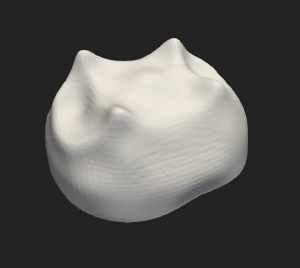# Results

An atlas consists in a mean shape and each deformation from the mean shape to each sample. Several outputs are written by Deformetrica:

• DemiSphere_template.vtk: vtk format surface. This is the final mean shape. Our mean shape:

• CP_final.txt : control point coordinates. Deformation are computed on a regular 3D grid located around the shapes and the locations of this points are optimised during the computation. These are the control points (with the first specimen):

• MOM_final.txt : momenta file, i.e. the parameters of the deformation for each specimen. Momenta vectors for the deformation from the mean shape to the first specimen (vector magnitudes are not to scale):

• DemiSphere_to_subject_X__t_Y.vtk : surfaces. For each X specimen, there are 20 surfaces which allow to visualise the deformation from the mean shape to the specimen. Example of deformation (mouse over to see animation):